#### Subject 6. Equilibrium GDP and Prices

Short-run macroeconomic equilibrium occurs when the quantity of real GDP demanded equals the quantity of real GDP supplied at the point of intersection of the AD curve and the SAS curve. If real GDP is below equilibrium GDP, firms increase production and raise prices, and if real GDP is above equilibrium GDP, firms decrease production and lower prices. These changes bring a movement along the SAS curve towards equilibrium.

In short-run equilibrium, real GDP can be greater than or less than potential GDP.

Long-run macroeconomic equilibrium occurs when real GDP equals potential GDP - when the economy is on its LAS curve.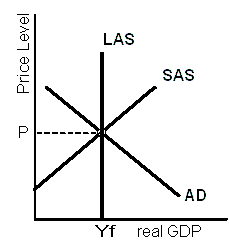Note two things:

• At the price chosen by suppliers (P), the aggregate demand (AD) is exactly equal to the amount suppliers are willing to supply (SAS).
• This equilibrium between SAS and AD coincides with the maximum capacity of the economy, as indicated by the LAS curve. The level of output produced is labeled as Yf, indicating full employment of resources.

Long-run equilibrium thus occurs where LAS, AD, and SAS coincide.

Economic Growth and Inflation

Economic growth occurs because the quantity of labor grows, capital is accumulated, and technology advances, all of which increase potential GDP and bring a rightward shift of the LAS curve. The following figure illustrates economic growth and inflation.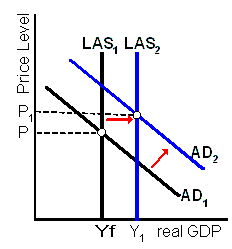Inflation occurs because the quantity of money grows faster than potential GDP, which increases aggregate demand by more than long-run aggregate supply. The AD curve shifts rightward faster than the rightward shift of the LAS curve.

The business cycle occurs because aggregate demand and short-run aggregate supply fluctuate.

• A below full-employment equilibrium is an equilibrium in which potential GDP exceeds real GDP. The amount by which potential GDP exceeds real GDP is called a recessionary gap.
• Long-run equilibrium is an equilibrium in which potential GDP equals real GDP.
• An above full-employment equilibrium is an equilibrium in which real GDP exceeds potential GDP. The amount by which real GDP exceeds potential GDP is called an inflationary gap.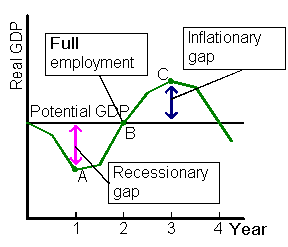Let's look at the inflation gap.

An economic boom may be the result of an increase in AD. Starting at long-run equilibrium, an increase in aggregate demand shifts the AD curve rightward.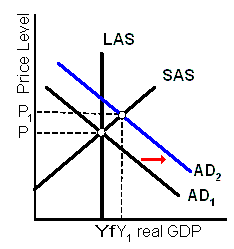The prices of goods and services increase, which in turn induces suppliers to expand output to a level that is unsustainable in the long run (which is why a boom is followed by an economic contraction). That is, firms increase output and prices - a movement along the SRAS curve.

Since prices are currently high (P1) and the situation is moving into the long run, people will expect prices to continue to be high. There is an inflationary gap.

Stagflation

In the resource market, a supply shock such as a drought or high oil prices is reflected by a leftward shift of the supply curve of resources. The price of resources, and thus the cost of production, increases. Assuming prices in the goods and services markets are unchanged, the higher costs may be one of the factors that contribute to a recession.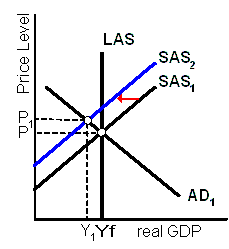As the SAS curve shifts leftward, real GDP decreases and the price level rises. The combination of recession with inflation is called stagflation.